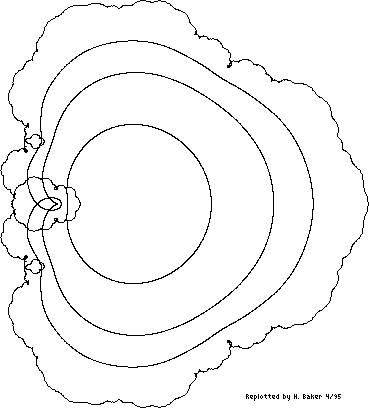Up

Figure 6(a). [Item 123] Image of circles |z| = 1/2, 3/4, 7/8, 1 under the function

```                      inf
===    n!
\     z
f(z) =  >    --- .
/     n!
===
n=1
```Figure 6(a). [Item 123] Image of circles |z| = 1/2, 3/4, 7/8, 1 under the function

```                      inf
===    n!
\     z
f(z) =  >    --- .
/     n!
===
n=1
```
[Retyped and formatted in html ('Web browser format) by Henry Baker, April, 1995.]